# Number theory derivation from infinity; speculations on equations that are derived in terms of the Field

## Recommended Posts

Let me repeat a previous post.

On 8/14/2021 at 12:50 PM, studiot said:

I should alsao like to point out that what Mathematicians mean by the word 'Field', particularly in relation to number theory,

and what Physicists mean are quite different.

You should disentangle the Physics and the Mathematics please.

OK so to continue with the Mathematics, though Euler also had much to do with applied maths.

Princeton University has been home to some of the greatest geniuses in History. They also have a small publishing house which publishes specialist topic books, most of which become standards in their field (see another use of the word field ?).

I am recommending one about Euler and his constant, gamma to you as you should find much of interest in it.
Most of the book is lightly mathematical to be more generally accessible but read the introduction here and see what you think.

Gamma - Exploring Euler's Constant

Julian Havil   -  Princeton University Press 2003 & 2021

##### Share on other sites

I would follow @studiot's advice here to disentangle physics and mathematics. There is a relationship to be sure, but there are many dangers in thinking one is the other.

As to the virial theorem, in case you're interested, I'm going to follow Studiot's example too and give you a piece of a very commendable book. Landau-Lifshitz, Course of Theoretical Physics, Vol. 1. Mechanics: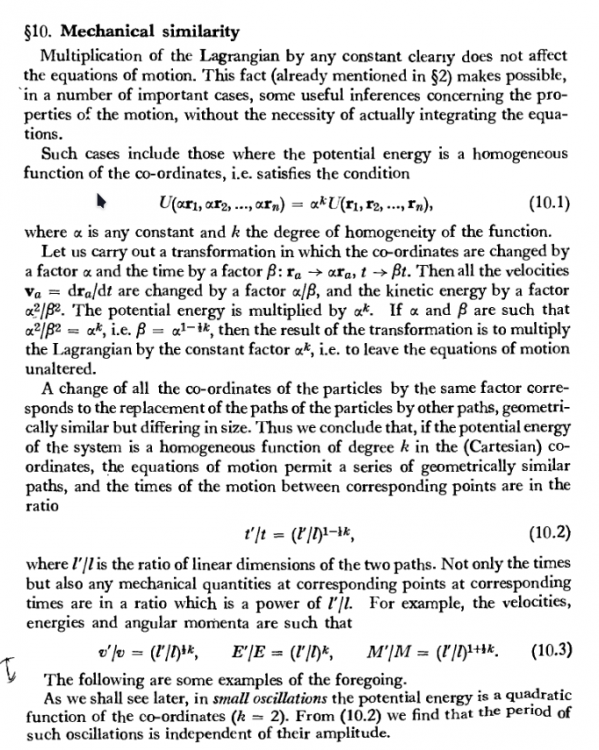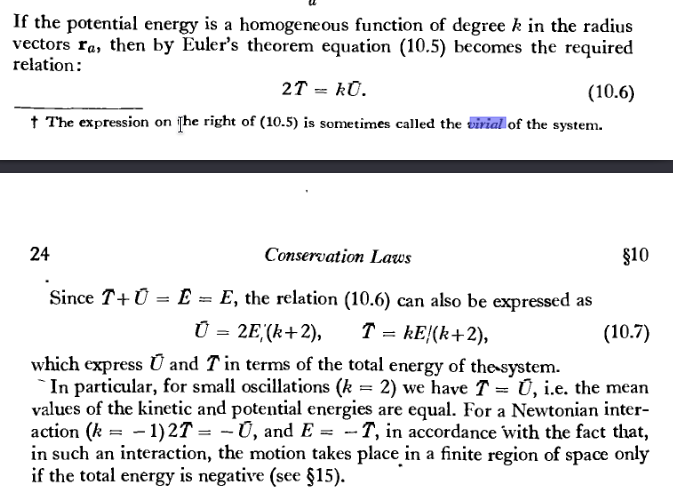##### Share on other sites

9 hours ago, studiot said:

Let me repeat a previous post.

On 8/14/2021 at 4:50 AM, studiot said:

I should alsao like to point out that what Mathematicians mean by the word 'Field', particularly in relation to number theory,

and what Physicists mean are quite different.

You should disentangle the Physics and the Mathematics please.

OK so to continue with the Mathematics, though Euler also had much to do with applied maths.

Princeton University has been home to some of the greatest geniuses in History. They also have a small publishing house which publishes specialist topic books, most of which become standards in their field (see another use of the word field ?).

I am recommending one about Euler and his constant, gamma to you as you should find much of interest in it.
Most of the book is lightly mathematical to be more generally accessible but read the introduction here and see what you think.

Gamma - Exploring Euler's Constant

Julian Havil   -  Princeton University Press 2003 & 2021

[...]

It bears repeating.

To highlight a math aspect: do you see any new applications vis vis ζ(1) or ζ    ?(x)

5 hours ago, joigus said:

I would follow @studiot's advice here to disentangle physics and mathematics. There is a relationship to be sure, but there are many dangers in thinking one is the other.

As to the virial theorem, in case you're interested, I'm going to follow Studiot's example too and give you a piece of a very commendable book. Landau-Lifshitz, Course of Theoretical Physics, Vol. 1. Mechanics:

It bears repeating.

I am missing (10.5 and likely more) but it was worth it finding F-E equation& totient theorem/function, for myself for study.

The mechanics bearing transformatinos giving ratios for velocities a/B, T (K.E.) a^2/B^2, U (P.E.) * a^k , I think I understand. Although k is fuzzyto me as degree of homogeneity (e.g. ideal gas law assumptions? uniform distribution in closed space?). We get to where we are transforming (conserving?) velocities, energy, and angular momentum, but here again what looks like
t'/t = (l'/l)^1-(1/2)k, then v'/v=(l'/l)^(1/2)k, E'/E=(l'/l)^k, M'/M=(l'/l)^(1+(1/2)k) with l'/l = the transformed path over the original in linear dimensions

k in the exponent again looks fuzzy. Of course, we want to conserve these classical physics mechanics...

they fit the virial theorem, and at the end of Ch. 10 as cited we have how they behave in a finite region of space if the total energy is 'negative' (see Ch. 15). Or, before that:  2T = kU  since T+U = E, by (10.6) U = 2 E / (k+2); T = -kE(k+2) , using averages for T&U and equating avg. E and E proper. I'll not pretend to actually understand the 1 modulo n from Euler's "totatives" business (wow...) but I made a long sidebar in the midst of my notes.

Our virial for chemistry appears to me to be broken...

Quote

In chemistry and quantum physics, quantum numbers describe values of conserved quantities in the dynamics of a quantum system. Quantum numbers correspond to eigenvalues of operators that commute with the Hamiltonian—quantities that can be known with precision at the same time as the system's energy[note 1]—and their corresponding eigenspaces. Together, a specification of all of the quantum numbers of a quantum system fully characterize a basis state of the system, and can in principle be measured together.

Gentlemen, I do not think I am entangled in maths and physics. We need a qay to quantize thosesmall oscillations in atoms/molecules, and the giant forces in particle physics (I think, but I don't know about that). My workup here, however, properly edited, I think is only looking at some math, and quantum chemistry. I do not think there is any appeal to physics exactly -- although the tie-in wit the Hamiltonian isthere... Much like with how much i (imaginary unit) is tied in all over the quantum math. How can we quantize both small and large??? I don't know how to do it, but I think i'm formulating the problem or question at hand properly. Corrections or other ideas of course are welcome and thank you again for prompting more work with yours.a

Edited by NTuft
teasing out earlier part of virial ; note*: independence from amplitude (apply to unit circle of complex polar graph)
##### Share on other sites

On 4/30/2022 at 5:20 PM, Genady said:

Successfully solving a problem that does not exist to start with. The solution is beautifully clear:

"the mathematical reflection of the dialectical law of affirmation-negation for qualitatively opposite binary judgments about the nature of any object or process."

Hello @Genardy ,
What a difficult to inpetret statement!  What do you mean by the first sentence??? there is a problem with quantization is it not?

On 4/30/2022 at 5:20 PM, Genady said:

"the "imaginary" unit i is a symbol for a qualitatively polar opposite essence (property, number, parameter) obeying to the polar opposite (“negative”) algebra of signs relative to the conventional (“positive”) algebra."

In conclusion,

"we believe that the word "imaginary" as not reflecting reality will eventually be removed from mathematics and physics, harmonizing the mathematical structures with the laws of the Universe, and thus expanding the horizons of knowledge."

@GenadyI took you quoting this (and all you said) to mean you enjoyed [ed: read*] the paper?? What did you think of it? Do you know about electrostatics?
Please, your response is very cryptic, I congratulate you. Can I thank you for reading that paper?

Edited by NTuft
##### Share on other sites

1 minute ago, NTuft said:

Hello @Genardy ,
What a difficult to inpetret statement!  What do you mean by the first sentence??? there is a problem with quantization is it not?

@GenadyI took you quoting this (and all you said) to mean you enjoyed the paper?? What did you think of it? Do you know about electrostatics?

Thank you for your interest. However, I don't intent to participate in this discussion any more.

+

-

##### Share on other sites

defined as the set of square roots of prime numbers, +&-. Inoperable set. -1 and +1 not prime...

derivation produces

produces

Derivation can continue.

The  level of complexity is characterized as AdS 4 space.

The 3-D  can be mapped with Quaternion/Octonion algebra.

2-D map on hyperbolic Poincaré disk, or using polar graph, as extension from

1-D "string" (or + - point charge seperation) causes initial planar geometry.

The nature of  can facilitate quantization at quantum level in the distribution around 0 and as distance increases up to the... Macroscopic.

Edited by NTuft
##### Share on other sites

9 minutes ago, NTuft said:

defined as the set of square roots of prime numbers, +&-. Inoperable set. -1 and +1 not prime...

derivation produces

produces

Derivation can continue.

Please elaborate on what you mean by these statements, since they are not mathematical ones I recognise.

Note mathematical statements need not be symbolic, English is perfectly good.

Please pay particular attention to what you mean by 'derivation' and 'set'.

I seen no point worrying about the rest of the material in your post until the basics are cleared up.Set Thoth

##### Share on other sites

3 hours ago, NTuft said:

Set Thoth

If you are not going to take the discussion seriously, you will need to find another to continue the discussion with.

##### Share on other sites

6 hours ago, studiot said:

I seen no point worrying about the rest of the material in your post until the basics are cleared up.

Use the fundamental theorem of arithmetic to make the top-down set.

2 hours ago, studiot said:

If you are not going to take the discussion seriously, you will need to find another to continue the discussion with.

I will try to formulate it properly. In this case I'm using it as the operation whereby the power (dimension) is reduced by 1 and that is brought down to a co-efficient.

##### Share on other sites

Dear @studiot,

On 5/3/2022 at 4:20 AM, studiot said:

Please elaborate on what you mean by these statements, since they are not mathematical ones I recognise.

Note mathematical statements need not be symbolic, English is perfectly good.

Please pay particular attention to what you mean by 'derivation' and 'set'.

From page 1:

Quote

I understand a derivative to be a measure of infinitesimal change at a point.  [...]

I think that this is the purpose of set theory: numbers are what we commonly refer to as the collection of objects [...]

These are my working definitions, and I know they're not correct, so I will look to redefine these terms properly, but, please do impart directly what you think is the most correct or necessary conditions/definitions for the purpose here, if you see fit.

Quote

since they are not mathematical ones I recognise.

I am not able to make LaTeX work properly yet, but I'm reading through that information.

derivation produces

produces:

I apologize, i do take this seriously. And no, I cannot define all my terms properly, I'll work on it. Your participation is appreciated but is up to you.

Thanks

Edited by NTuft
formatting
##### Share on other sites

I understand derivation to mean a series of steps leading to a statement or conclusion.

I think you mean 'differentiation' which means calculating the derivative as per the differential calculus.

I know that in some other disciplines differentiation means distinguishing between alternatives.

You differentiate a function and the result is known as the derived function or the derivative.

Spooky.

Life is made more difficult because you can't 'differentiate' a point or single member of a set or a set itself.

This is my objection to your lines

derivation produces

produces:

since you have already defined i' as a set.

Does this help ?

##### Share on other sites

Quote

In mathematics, a set is a collection of elements. The elements that make up a set can be any kind of mathematical objects: numbers, symbols, points in space, lines, other geometrical shapes, variables, or even other sets. The set with no element is the empty set; a set with a single element is a singleton. A set may have a finite number of elements or be an infinite set. Two sets are equal if and only if they have precisely the same elements.

derivative: https://handwiki.org/wiki/Derivative

Quote

[...]the derivative is often described as the "instantaneous rate of change", the ratio of the instantaneous change in the dependent variable to that of the independent variable.

differential: https://handwiki.org/wiki/Differential_(mathematics)

Quote

In mathematics, differential refers to infinitesimal differences or to the derivatives of functions.

Quote

In mathematics, a function is a binary relation between two sets that associates each element of the first set to exactly one element of the second set. Typical examples are functions from integers to integers, or from the real numbers to real numbers.

differential operator: https://handwiki.org/wiki/Differential_operator

Quote

In mathematics, a differential operator is an operator defined as a function of the differentiation operator. It is helpful, as a matter of notation first, to consider differentiation as an abstract operation that accepts a function and returns another function (in the style of a higher-order function in computer science).

it'd seem to establish it as a function would only require that the set be mapped to another set. as a function it could then be differentiated, producing the derivatives as proposed. what this entails, i do not know -- it could be a nothing burger.

or, it could be a Hamiltonian operator: the potential number of values that can be assigned to objects (particles) as kinetic(i'') or potential(i''') energy are unlimited, and it seems to me a 'gapped hamiltonian' in that the values are discrete with 'jumps' in between. just more speculations.

Edited by NTuft

(+)(+)

(-)(-)

(+)(-)

(-)(+)

Edited by NTuft
##### Share on other sites

7 hours ago, NTuft said:

scratch that last.

Where  = (i'+)(i'-)=(1.4142...)(-1.4142...),

(i'+)cos()+ (i'-)sin() = cos()+ sin()=()(-1) + ()(0) = -  ;

##### Share on other sites

…none of this is accurate, and this can be checked directly by e.g. wolfram alpha.

e^(i’ pi) = e^ (i sqrt(2) pi) = cos(sqrt(2) pi) + i sin(sqrt(2) pi) ~ -0.266 - 0.963 i

##### Share on other sites

On 5/8/2022 at 6:46 AM, NTuft said:

it'd seem to establish it as a function would only require that the set be mapped to another set. as a function it could then be differentiated, producing the derivatives as proposed. what this entails, i do not know -- it could be a nothing burger.

I really don't see where you are trying to get to.

A function has three parts so you are right in saying that a function is a map from one set to another (which may be the same set).

The base set or set mapped from is called the Domain and the target set or set mapped to is called the CoDomain.

A function comprises the Domain, the CoDomain and a rule that links members of the Domain to members of the CoDomain.

Many (or even all) members of the Domain may be linked to some particular member of the CoDomain, this is called many-to-one.

But no member of the Domain may be linked to more than one member of the Codomain.  This would called one-to-many and is not allowed.

A function that links all the members of the domain to one single member of the CoDomain is called a constant function.

It is better to think of the three components as being two variables (say x and y since we are discussing numbers)  and a rule or formula connecting them.

The Domain is called the independant variable (x) and the CoDomain is called the dependant variable (y).

For most purposes the Domain will be the set of real numbers or the real number line.

The constant function has the rule y = a constant, say y = 5.

This means that for each and every value of x the value of y is 5.

This function can indeed be differentiated and the value of its derivaitve for all values of x is zero.

I hope you will find this little exposition helpful and will listen to it.

##### Share on other sites

2 hours ago, uncool said:

…none of this is accurate, and this can be checked directly by e.g. wolfram alpha.

e^(i’ pi) = e^ (i sqrt(2) pi) = cos(sqrt(2) pi) + i sin(sqrt(2) pi) ~ -0.266 - 0.963 i

when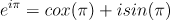=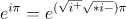=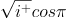+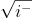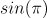= (+1)([cos(pi)=-1]) + (-1)([sin(pi)=0]) = (+1)(-1) + (-1)(0) = -1

I think the positive co-efficient (+1) is there in front of cosine, though iis not denoted--since it's the "real" half of the root of -1

-----

using

(+1.4142...)(+1.4142...)

(-1.4142...)(-1.4142...)

(+1.4142...)(-1.4142...)

(-1.4142...)(+1.4142...)

now with  = (i'+)(i'-)=(+1.4142...)(-1.4142...),

(i'+)cos()+ (i'-)sin() = cos()+ sin()=()(-1) + ()(0) = -  ;

since that co-efficient in front of cosine is now

Edited by NTuft
##### Share on other sites

Oh dear, Oh dear, Oh dear.

You should have believed me when I strongly suggested you took notice of what uncool says.

2 hours ago, uncool said:

none of this is accurate,

Right at the beginning.

27 minutes ago, NTuft said:

when

The square root of something is  defined as that which which when multiplied by itself yields that very something.

But multiplying the suqare root of minus one by the square root of plus one is not multiplying something by itself, since the square root of mius one is not the same as the square root of plus one.

Edited by studiot
##### Share on other sites

…no, NTuft, that is not why e^(i pi) = -1. Your blind substitution is not correct.

You are claiming that e^(x*i*pi) = x cos(pi) + i x sin(pi) = -x, if I’ve remade your equations correctly. This simply isn’t true. It has nothing to do with the actual justification of the original equation (which has to do with McLaurin series), and is self-contradictory with a bit of thought.

##### Share on other sites

6 hours ago, studiot said:

The square root of something is  defined as that which which when multiplied by itself yields that very something.

There is a problem with defining the square roots of negative numbers, in my opinion. I went over how I wanted to do that. There are two ways to do it for positive numbers, and I think there are two ways to do it for negative numbers.

Also, the explanation on the domain set being mapped onto the codomain by a function, and the many-to-one vs. one-to-many rules are helpful, thank you. I will try to wrap my head around it... Sorry, but I think it will help.

4 hours ago, uncool said:

…no, NTuft, that is not why e^(i pi) = -1. Your blind substitution is not correct.

You are claiming that e^(x*i*pi) = x cos(pi) + i x sin(pi) = -x, if I’ve remade your equations correctly. This simply isn’t true. It has nothing to do with the actual justification of the original equation (which has to do with McLaurin series), and is self-contradictory with a bit of thought.

Hi uncool.

I do not think you have what I am claiming figured. I meant to illustrate in the post I quoted you in above that the numbers you ran on WA were not what I was implying. In the second iteration, still yet, no -- operative is:  "with ". Then the imaginary number in the exponent is now that one I've just set, which I then split.

I will definitely look into how the McLaurin series is tied in and I appreciate your knowledge about (I imagine) sequences, series(just mentioned), sets (I know), and the postulates, forms, rules and proofs that go into that branch of math, which I bet you know well -- it's not my strong suit.

--

"e^(x*i*pi) = x cos(pi) + i x sin(pi) = -x,"

x=pi already in that equation, no?

Edited by NTuft
##### Share on other sites

6 hours ago, NTuft said:

There is a problem with defining the square roots of negative numbers, in my opinion. I went over how I wanted to do that. There are two ways to do it for positive numbers, and I think there are two ways to do it for negative numbers.

There is no problem with defining 'square roots'.

Problems do arise when failing to distinguish between the square root function and a specific square root, just as with any function it is necessary to distinguish between the function itself and the value of that function at a particular point, often called 'the square root of ...'

I have already defined a square root - and this applies equally well to negative as to positive numbers and to zero, which is neither positive nor negative.

13 hours ago, studiot said:

The square root of something is  defined as that which which when multiplied by itself yields that very something.

Now consider the number 4.

My definition is satisfied by two different numbers  +2  &  -2.

I hope we can agree that these are different.

so multiply (+2) x (+2)    or (-2)  x (-2)

Both of these products yield the initial value of 4, as per definition.
So both are square roots.

But what happens if you multiply one by the other ?

(-2) x (+2)    or (+2)  x (-2)

Neither are equal to the initial value of +4 !

You cannot take the negative sign inside the square root sign, like you do with brackets, which is what you appear to be trying to do.

$- \sqrt 4 = \sqrt { - 4}$

is just plain wrong  - it is never right.

7 hours ago, NTuft said:

Also, the explanation on the domain set being mapped onto the codomain by a function, and the many-to-one vs. one-to-many rules are helpful, thank you. I will try to wrap my head around it... Sorry, but I think it will help.

Many problems with functions arise because people only consider the rule and forget to consider the Domain and/or the CoDomain as well.
This consideration is necessary when considering the whole function.

##### Share on other sites

I have always thought of there being parentheses inside the root exponential function.

I would argue that the numbers are opposite in magnitude but are the same number, precisely.

It may be that I was only trying to find the unit hyperbola, or the complex hyperbolic space.

Quote

Parametrization:

A direct way to parameterizing the unit hyperbola starts with the hyperbola xy = 1 parameterized with the exponential function:

.

This hyperbola is transformed into the unit hyperbola by a linear mapping having the matrix:

This parameter t is the hyperbolic angle, which is the argument of the hyperbolic functions.

One finds an early expression of the parametrized unit hyperbola in Elements of Dynamic (1878) by W. K. Clifford. He describes quasi-harmonic motion in a hyperbola as follows:

Quote

The motion  has some curious analogies to elliptic harmonic motion. ... The acceleration thus it is always proportional to the distance from the centre, as in elliptic harmonic motion, but directed away from the centre.

I thought perhaps the vertical (lower, or limit) bounds for the complex graph should be  , and the horizontal perhaps  when , which would make an ellipse, so the unit hyperbola scaled differently and now with an ellipse...

but of course we can not screw up hyperbolic functions:

Quote

is half the difference of  and

is the average of  and

It may be we can already do everything we need to with , but it seems clunky to me...

I doubt this is correct or useful either but I had it worked out we could quantize Kinetic Energy with the bound that makes .5 from the function,  , or -- set that limit velocity as equal to the mass of the proton.

I had Potential Energy for, I imagine, an electron  whereby two electrons at (-.25) balance a proton charge (possibly confounding charges and energies...), but, with TI-83 (handheld scientific calculator) I had the P.E. function increasingly negatively (as though the electron were further from the proton), whereas the KE function  set an upper .5 bound with  and was going towards a limit of 0. I cannot re-create the numbers the same for PE through WolframAlpha computation, and I'm not sure what the exponent should be for PE (3/2, 1.5,...).

I will try to only "crank" out what is necessary for this thread. I would not rule out that

On 5/11/2022 at 2:20 AM, studiot said:

There is no problem with defining 'square roots'.

I thought the "imaginary unit" was kind of clunky, and we could somehow give magnitude to complex numbers if we define the i as being members of set. But, maybe we can do everything with i being the multiplier that signifies a negative root.

Lastly I don't know if I can use +1 and -1 as arguments in the function given current definitions, but iterations of +1's and -1/2's for protons and electrons respectively I thought made sense some how.

Edited by NTuft

## Create an account

Register a new account